Processing ......FreeComputerBooks.com Free Computer, Mathematics, Technical Books and Lecture Notes, etc.

Computational and Algorithmic Mathematics. Symbolic ComputationRelated Book Categories:
•Mathematics in the Age of the Turing Machine (Thomas C. Hales)

Computers have rapidly become so pervasive in mathematics that future generations may look back to this day as a golden dawn. The article gives a survey of mathematical proofs that rely on computer calculations and formal proofs.

•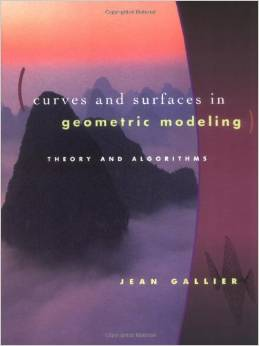Curves and Surfaces in Geometric Modeling: Theory and Algorithms

This book offers both a theoretically unifying understanding of polynomial curves and surfaces and an effective approach to implementation. It is also an exellent introduction to geometry concepts used in computer graphics, vision, robotics, geometric modeling.

•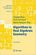Algorithms in Real Algebraic Geometry (Saugata Basu, et al)

The monograph gives a self-contained detailed exposition of the algorithmic real algebraic geometry. It will be useful both for beginners and for advanced readers, who work in real algebraic geometry or apply its methods in other fields.

•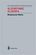Algorithmic Algebra (Bhubaneswar Mishra)

Anyone need to use algorithms in polynomials should have a copy of this! This is definitely one of the best! It covers almost everything anyone need in computation with systems of polynomial equations: Grobner Basis, Resultants, Characteristic Set, etc.

•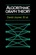Algorithmic Graph Theory (David Joyner, et al)

This is an introductory book on algorithmic graph theory. Theory and algorithms are illustrated using the Sage open source mathematics software. It's especially suitable for computer scientists and mathematicians interested in computational complexity.

•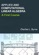Applied and Computational Linear Algebra: A First Course

This book is intended as a text for a graduate course that focuses on applications of linear algebra and on the algorithms used to solve the problems that arise in those applications.

•Shape Interrogation for Computer Aided Design and Manufacturing

This book provides the mathematical fundamentals as well as algorithms for various shape interrogation methods including nonlinear polynomial solvers, intersection problems, differential geometry of intersection curves, distance functions etc.

•LEDA: A Platform for Combinatorial and Geometric Computing

LEDA is a library of efficient data types and algorithms and a platform for combinatorial and geometric computing. This book, written by the main authors of LEDA, is the definitive account of how the system operates and how it can be used.

•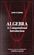Algebra: A Computational Introduction (John Scherk)

This book is a unique approach and presentation, the author demonstrates how software can be used as a problem-solving tool for algebra.

•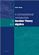A Computational Introduction to Number Theory and Algebra

This introductory book emphasises algorithms and applications, such as cryptography and error correcting codes, and is accessible to a broad audience.

•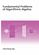Fundamental Problems of Algorithmic Algebra (Chee-Keng Yap)

This book provides a systematic and focused treatment of a collection of core problemsthe computational equivalents of the classical Fundamental Problem of Algebra and its derivatives.

•Algorithmic Number Theory: Lattices, Curves, and Cryptography

This text provides a comprehensive introduction to algorithmic number theory for beginning graduate students, written by the leading experts in the field.

•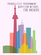Probabilistic Programming and Bayesian Methods for Hackers

This book is designed as an introduction to Bayesian inference from a computational understanding-first, and mathematics-second, point of view. The book assumes no prior knowledge of Bayesian inference nor probabilistic programming.

•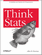O'Reilly® Think Stats: Probability and Statistics for Programmers

This book shows you how to perform statistical analysis computationally, rather than mathematically, with programs written in Python.

•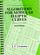Algorithms for Modular Elliptic Curves, 2nd Edition (J. E. Cremona)

This book presents a thorough treatment of many algorithms concerning the arithmetic of elliptic curves, with remarks on computer implementation. An extensive set of tables is provided giving the results of the author's implementation of the algorithms.

•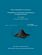From Algorithms to Z-Scores: Probabilistic and Statistical Modeling

This is a textbook for a course in mathematical probability and statistics for computer science students.

•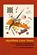Algorithmic Game Theory (Noam Nisan, Tim Roughgarden, et al)

This book covers many of the hottest area of useful new game theory research, introducing deep new problems, techniques, etc.

•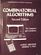Combinatorial Algorithms for Computers and Calculators (H. S. Wilf)

On one level, this is a collection of subroutines, in FORTRAN, for the solution of combinatorial problems.

•Combinatorial and Computational Geometry (Jacob E. Goodman)

It includes surveys and research articles exploring geometric arrangements, polytopes, packing, covering, discrete convexity, geometric algorithms and their complexity, and the combinatorial complexity of geometric objects, particularly in low dimension.

•Handbook of Computational Statistics: Concepts and Methods

This handbook cover the important subareas of computational statistics and give some flavor of the wide range of applications.

•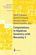Computations in Algebraic Geometry with Macaulay 2 (D.Eisenbud)

This book presents algorithmic tools for algebraic geometry and experimental applications of them using Macaulay 2.

•Matters Computational: Ideas, Algorithms, Source Code

This book provides algorithms and ideas for computationalists, whether a working programmer or anyone interested in methods of computation.

•Art Gallery Theorems and Algorithms (Joseph O'Rourke)

This book is the most comprehensive collection of results on polygons currently available and thus earns its place as a standard text in computational geometry.

•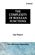The Complexity of Boolean Functions (Ingo Wegener)

Initially deals with the wee-known computation models, and goes on to special types of circuits, parallel computers, and branching programs.

•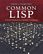Common LISP: A Gentle Introduction to Symbolic Computation

This highly accessible introduction to Lisp is suitable both for novices approaching their first programming language and experienced programmers interested in exploring a key tool for artificial intelligence research.

•Zen-Style Programming (Nils M. Holm)

This book introduces the concepts of functional and symbolic programming, applies these techniques to some problems of varying complexity.

•Strange Attractors: Creating Patterns in Chaos (Julien C. Sprott)

This book describes a simple method for generating an endless succession of beautiful fractal patterns by iterating simple maps and ordinary differential equations with coefficients chosen automatically by the computer.

•Computational and Algorithmic Mathematics

This is the previous page of Computational and Algorithmic Mathematics, we are in the processing to convert all the books there to the new page. Please check this page daily!!!

Book CategoriesAll CategoriesRecent BooksIT Research LibraryMiscellaneous Books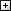Computer LanguagesComputer ScienceData Science/DatabasesElectronic EngineeringJava and Java EE (J2EE)Linux and UnixMathematicsMicrosoft and .NETMobile ComputingNetworking and CommunicationsSoftware EngineeringSpecial TopicsWeb Programming
Other Categories